# Ratio Scale

Here we will learn about a ratio scale, including using scale factors, scale diagrams and maps. We will also look at lengths, areas and volumes.

There are also ratio scale worksheets based on Edexcel, AQA and OCR exam questions, along with further guidance on where to go next if you’re still stuck.

## What is ratio scale?

Ratio scale is a proportional relationship between two quantities. These quantities are usually length, area, and volume but can also include population, frequencies, temperature and money.

A model aircraft is created by taking the width of the real object and reducing the size of it using a scale factor. A scale factor is a number which is used to describe an enlargement.

We also have to consider the units for this example. Here, the original object is 15m wide, which is equal to 1500cm. The ratio of the model to the original (in centimetres) is 50:1500 which simplifies to 1:30.

So the ratio of the model to the real object would be 1:30 as the real object is 30 times larger than the model.

Top tip: The ratio scale is usually given in the form 1:n where n is the scale factor of the enlargement ( n can be positive or negative).

There are multiple topics that require a scale factor or a ratio scale to fulfil the specific criteria of the question.

### What is ratio scale?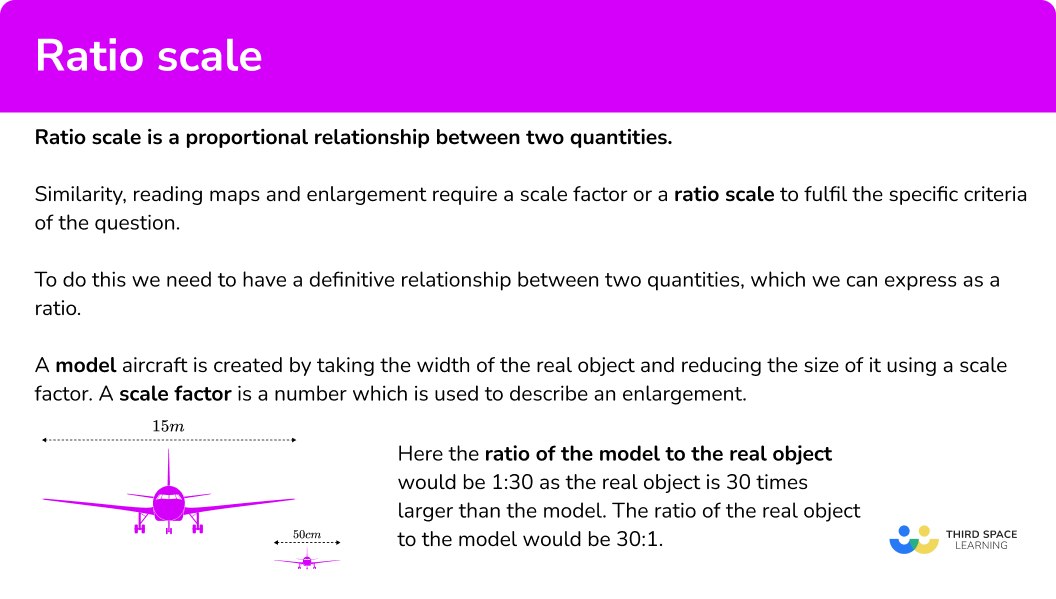• Maps

We can use a ratio scale to work out the actual distances represented on a map.

For example,

A map has a scale of 1:20 \ 000 (read as 1 to 20 \ 000 ). This means that for every 1cm distance measured on the map, the real life value would be 20 \ 000 times greater.

If we measure the distance between the Post Office and the Supermarket, we measure 4.2cm. The real / actual distance is therefore 4.2 \times 20 \ 000=84 \ 000 \ cm. To convert this to metres, we need to divide the distance by 100,

84 \ 000 \div 100=840m .

So the Supermarket is 840m away from the Post Office.

• Length, area and volume

We can also use a ratio scale to compare the length, area and volume of shapes.

Let’s look at two similar 3D shapes.

Here we have two similar cuboids

The lengths of cuboid A have been multiplied by a scale factor of 2.

We call this the length scale factor or LSF . The ratio of the lengths of A to B is 1:2 .

If we calculate the area of the front face rectangles we can find the area ratio.

Cuboid A ’s front face has an area of 3 \times 5 = 15 \; cm^2

Cuboid B ’s front face has an area of 6 \times 10 = 60 \;cm^2

The area of A has been multiplied by a scale factor of 4 to give the area of B.

We call this the area scale factor or ASF . In general ASF = LSF^2

The ratio of the areas of A to B is 1:4 .

If we calculate the volume of the cuboids we can find the volume ratio.

Cuboid A has a volume of 3 \times 5 \times 2 = 30 \; cm^3

Cuboid B has a volume of 6 \times 10 \times 4 = 240 \; cm^2

The volume of A has been multiplied by a scale factor of 8 to give the volume of B.

We call this the volume scale factor or VSF . In general VSF = LSF^3

The ratio of the volumes of A to B is 1:8 .

To summarise:

If we have any two similar shapes whose lengths are in the ratio a:b .

Their areas will be in the ratio a^2:b^2 .

Their volumes will be in the ratio a^3:b^3 .

### Bar modelling

Bar modelling can help for ratio scales because we can use the value of each share to determine a missing value. For example, below are a group of triangles arranged into a bar model.

If the total of the share value for \text{B} was 24 , what would the share value be for \text{A} ?

Each triangle has the same share value and so if we know the share value of one triangle, we can calculate the total of the share values for \text{A} .

24 \div 2=12 so each triangle is worth 12.

Writing this onto the diagram, we get

Adding up the total value of the shares for \text{A}, we get 12+12+12=12 \times 3=36.

Writing this as a ratio scale, we have used the ratio 3:2 to find the other value in the ratio. This can be useful for reading map scales, finding missing lengths or drawing scale diagrams.

We could also find the total amount by adding up all the shares together. Here, this would be 12 \times 5=60.

Step-by-step guide: Scale maths

## How to use a ratio scale

In order to use a ratio scale:

1. Write down two related quantities from the question / diagram.
2. Calculate the scale factor / ratio of the two quantities.
3. Use the scale factor to determine other scaled values.

### Explain how to use a ratio scale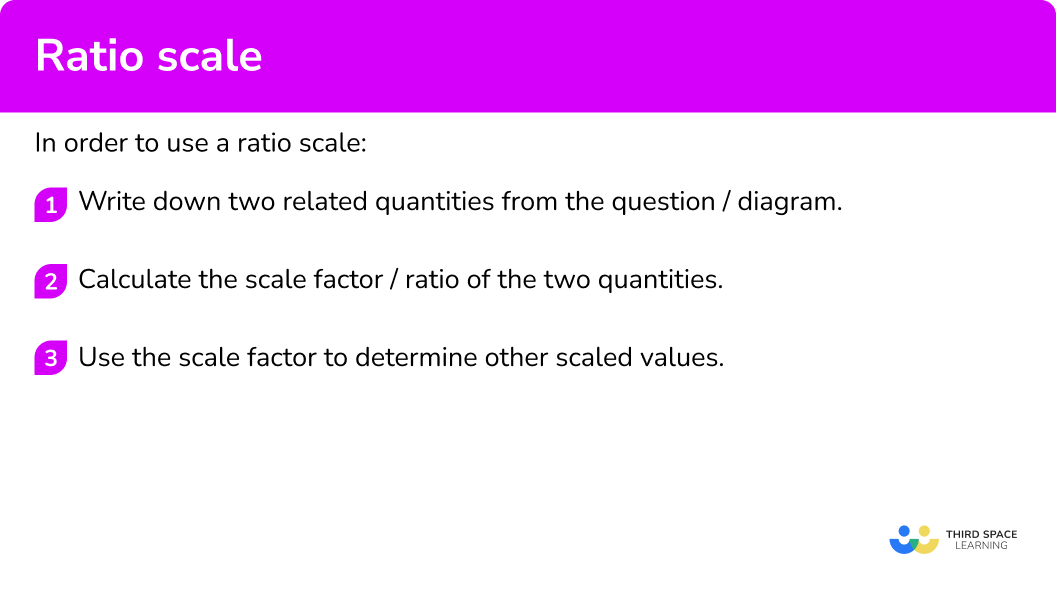## Related lessons on ratio

Ratio scale is part of our series of lessons to support revision on ratio. You may find it helpful to start with the main ratio lesson for a summary of what to expect, or use the step by step guides below for further detail on individual topics. Other lessons in this series include:

## Ratio scale examples

### Example 1: similar shapes

Below are two similar shapes. Use the information provided to calculate the value of x.

1. Write down two related quantities from the question / diagram.

We know the two lengths \text{AB} and \mathrm{A}^{\prime}\mathrm{B}^{\prime} and so we can write these as a ratio 5:45.

2Calculate the scale factor / ratio of the two quantities.

Simplifying the ratio 5:45 by dividing both sides by 5, we get the ratio 1:9.

This means that the length of \mathrm{A}^{\prime}\mathrm{C}^{\prime} is 9 times greater than the length of \text{AC} .

3Use the scale factor to determine other scaled values.

6 \times 9=54cm.

\mathrm{A}^{\prime}\mathrm{C}^{\prime}=54cm.

### Example 2: reading a map scale

A map has a scale of 1:50 \ 000. The distance between two points on a map is equal to 5.6cm. Calculate the real distance in kilometres.

As we have been given the ratio scale 1:50 \ 000 , we can move on to step 3 straight away.

Move on to Step 3.

Using equivalent ratios, we can state the following

We therefore need to find the value of x by calculating 50000 \times 5.6=280000.

Converting 28000cm to km, we get

### Example 3: scale model

A dinosaur bone measures 1.8m in length. Paula is producing a scale model of the dinosaur. A scale model of another bone measuring 0.96m in length, is 16cm long.

Calculate the length of the first bone for the model.

The quantities we can use are the lengths of the second bone. The actual length is 0.96m \ (96cm) and the model length is 16cm.

This gives us the ratio 96:16 which simplifies to 6:1. The model is 6 times smaller than the original dinosaur bone.

As the original bone is 1.8m \ (180cm) in length, the length of the scale model is equal to

180 \div 6=30cm .

### Example 4: standard form

The atomic radius of a magnesium atom is equal to 1.6 \times 10^{-8}cm. A centimetre is 10 \ 000 \ 000 nanometres. Calculate the atomic radius of one atom of magnesium in nanometres.

Here we are converting units of length from centimetres to nanometres. It states that 1cm is equal to 10 \ 000 \ 000nm.

The ratio to convert centimetres to nanometres is therefore 1:10 \ 000 \ 000. In standard form, this is equal to 1:1 \times 10^{7}.

The atomic radius of magnesium is 1.6 \times 10^{-8} and so by multiplying this by 1 \times 10^{7} we get

1.6 \times 10^{-8} \times 1 \times 10^{7}=1.6 \times 10^{-1}=0.16nm.

The atomic radius of magnesium is 0.16nm.

### Example 5: area

Below are two similar rectangles. The area of rectangle \text{A} is 12cm^{2}. The area of rectangle \text{B} is 108cm^{2}. If the width of rectangle \text{B} is 12cm, calculate the height of Rectangle \text{A} .

The two quantities we can compare are the cross sectional areas for each cuboid, 12cm^2 and 108cm^2.

12:108=1:9

As this is an area scale factor, we need to be careful with units. Here we have the ratio 1cm^{2}:9cm^{2}.

To get the linear scale factor, we need to square root both parts of the ratio to get the length scale factor. \sqrt{1}:\sqrt{9}=1:3 so we now have the ratio 1:3 for the lengths of the cuboid.

As the width of Cuboid \text{B} is 12cm, 108 \div 12=9cm, so the height of Cuboid \text{B} is 9cm.

We want to determine the value of x in the ratio x:9 (the heights of the cuboids).

The equivalent ratio we can use is the ratio 1:3 and so as 3 \times 3=9, 1 \times 3=x=3.

The height of Cuboid \text{A} is 3cm.

### Example 6: bar modelling

An amount of money is shared between Eddie and Fen in the ratio 7:2. If Fen received £36, how much did Eddie receive?

Using a bar model, we have

As Fen’s 2 parts are worth £36 we need to work out what one part is worth.

36 \div 2=18

Eddie has 7 parts, so receives £126.

18 \times 7=£126

### Common misconceptions

• Ratio scale used incorrectly

Make sure you have the ratio the correct way around. For example, the height of a scale drawing of a building is 0.32m. The actual height of the building is 96m.

The ratio scale of the drawing to the real building is therefore 0.32:96 = 1:300 so the real building is 300 times taller than the drawing, not the other way around.

• Converting units

The unit conversion is mixed up. For example, the ratio of the units of centimetres to millimetres is given as 10:1 whereas this should be 1:10 (there are 10mm in 1cm and so you must multiply cm by 10 to get mm ).

• Area and volume conversions

When given the area or volume of two shapes and you need to find a missing length, you need to determine the linear scale factor. This is done by finding the square root of the area scale factor or the cube root of the the volume scale factor respectively. For example, the two cuboids below are similar.

The volume scale factor would be equal to 60:480=1:8 whereas the length scale factor would equal \sqrt{1}:\sqrt{8}=1:2. This means that the volumes would be multiplied by 2^3 whereas the lengths are multiplied by 2. Furthermore, the surface area would be multiplied by 2^{2}.

### Practice ratio scale questions

1. Calculate the length of \text{AB}.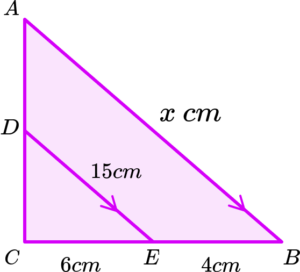10cm60cm25cm22.5cm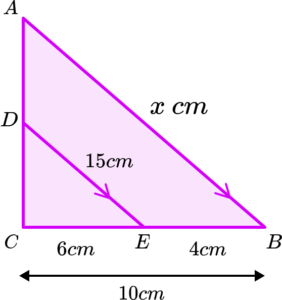\begin{aligned} &CE:CB=6:10=1:\frac{5}{3} \\\\ &AB=15\times\frac{5}{3}=25\text{cm} \end{aligned}

2. A map uses the scale 1:25 \ 000. Toby walked 20km. How far would this be on the map in centimetres?

5080500 \ 0005\begin{aligned} &25000cm=250m=0.25km \\\\ &20 \div 0.25=80cm \end{aligned}

3. A scale model of a block of flats is built. It uses the scale 1:200. If the height of the model is 60 \ cm , how tall is the real block of flats? Give your answer in metres.

12 \ 000120121 \ 200\begin{aligned} &60 \times 200=12 \ 000cm \\\\ &12 \ 000 \div 100=120 m \end{aligned}

4. A palisade cell is observed under a microscope. The length of the cell is 8µm ( 8 micrometres). 1 micrometre is ten thousandths of a centimetre.

The researcher draws a scale drawing. The length of the scale drawing is 4cm.

Calculate the ratio scale for the actual size to the scale drawing.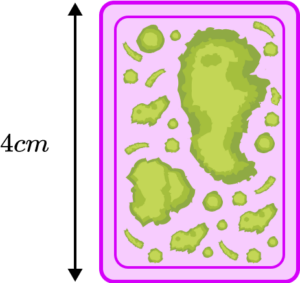1:500001:50002:11:32000\begin{aligned} &1µm=0.00001cm \\\\ &8µm=0.00008cm \\\\ &4 \div 0.00008=50000 \end{aligned}

5. Cube \text{A} has a surface area of 54cm^{2}. Cube \text{B} has a surface area of 96cm^{2}. Calculate the ratio scale of the side lengths of cube \text{A} to cube \text{B}.

9:1616:93:44:3Area

54:96=9:16

Length

\sqrt{9}:\sqrt{16}=3:4

6. An amount of sweets is shared between Adam and Bryn in the ratio 5:6. Adam gets 35 sweets, how many sweets are there altogether?

7774284\begin{aligned} &35 \div 5=7 \\\\ &6 \times 7=42 \\\\ &35+42=77 \end{aligned}

### Ratio scale GCSE questions

1. Below is a scale drawing map of a desert island.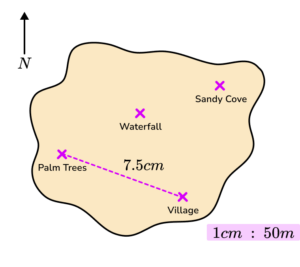(a) Calculate the actual distance between the Palm Trees and the Village in centimetres.

(b) Gareth says “the distance between the Palm Trees and the Waterfall is 25m ”. Explain why Gareth must be wrong.

(3 marks)

(a)

7.5 \times 50=375

(1)

37 \ 500 \ cm

(1)

(b)

The distance between the Palm Trees and the Waterfall is shorter than the distance between the Palm Trees and the Village.

Or

25m would only be 0.5 \ cm on the map, and the distance is longer than that

(1)

2. (a) Below are two similar isosceles triangles. Calculate the height of triangle \mathrm{A}^{\prime}\mathrm{B}^{\prime}\mathrm{C}^{\prime}.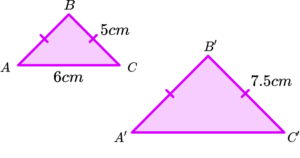(b) State the ratio of areas for triangles \text{ABC} to \mathrm{A}^{\prime}\mathrm{B}^{\prime}\mathrm{C}^{\prime}. Write your answer in its simplest form.

(6 marks)

(a)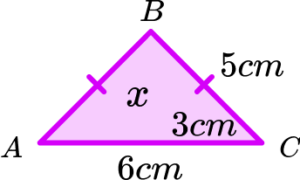Pythagoras’ theorem: x=\sqrt{5^{2}-3^{2}}=4\text{cm}

(1)

5:7.5=1:1.5

(1)

4 \times 1.5=6cm

(1)

(b)

12:27

(1)

4:9

(1)

3. The table below shows an estimate for the distance a planet in the solar system is from Earth on one specific day.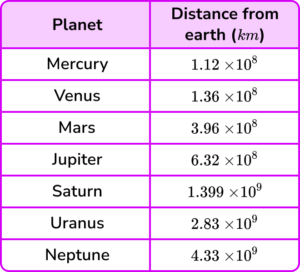Calculate the ratio scale of the distances of Neptune from Earth, to Mercury from Earth? Write your answer in the form 1:n.

(3 marks)

(4.33 \times 10^{9}):(1.12 \times 10^{8})

(1)

(1.12 \times 10^{8}) \div (4.33 \times 10^{9})

(1)

1:2.5866 \times 10^{-2}

(1)

## Learning checklist

You have now learned how to:

• Use scale factors, scale diagrams and maps
• Compare lengths, areas and volumes using ratio notation and/or scale factors – making links to similarity

## Still stuck?

Prepare your KS4 students for maths GCSEs success with Third Space Learning. Weekly online one to one GCSE maths revision lessons delivered by expert maths tutors.

Find out more about our GCSE maths tuition programme.